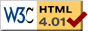### Journée-séminaire de combinatoire

#### (équipe CALIN du LIPN, université Paris-Nord, Villetaneuse)

Le 23 janvier 2018 à 14h00 en B107, Vincent Jugé nous parlera de : Uniform generation of infinite concurrent runs: the case of trace monoids

Résumé : We introduce an algorithm for the uniform generation of infinite runs in concurrent systems under a partial order probabilistic semantics. Using trace monoids as concurrency models, we will first focus on the notion of a uniform distribution on infinite traces in general and on the simulation of such a distribution. Then, we will introduce our algorithm, which aims at simulating a uniform distribution in a distributed context. This algorithm outputs on-the-fly approximations of a theoretical infinite run, the latter being distributed according to the exact uniform probability measure. The average size of the approximation grows linearly with the time of execution of the algorithm, and the execution of the algorithm only involves distributed computations, provided that some - costly - precomputations have been done. This presentation is based on a joint work with Samy Abbes.

 Dernière modification : Monday 24 January 2022Contact pour cette page : Cyril.Banderier at lipn.univ-paris13.fr